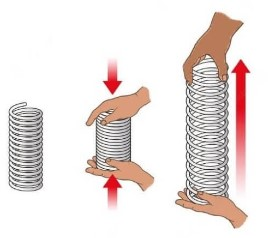# Elastic Potential Energy Calculator

To find unknown term of elastic potential energy, fill the input boxes and click calculate button

Formula:
U = 1/2 kx2
n/m
m

Give Us Feedback

The elastic potential energy (EPE) calculator finds the unknown value in the EPE formula. These values are:

• Spring potential energy
• Spring stretch length
• Spring force constant

It displays the formula for each value. To avoid any error in the result, the calculator has units written against the input boxes. This way the user will know which unit to use while entering the data.

## How to use the EPE calculator?

To use this tool:

1. Select the unknown value e.g Spring potential energy.
2. Enter the remaining two values.
3. Press Enter.

The result will appear right after you complete all of these steps. You can reset the tool. Talking of springs, also try Hooke’s law calculator.

## What is Elastic Potential Energy?

There are two main types of potential energy; Elastic and Gravitational potential energy. The potential energy which deals with stress and strain is called elastic potential energy.

When an object e.g a spring, is stretched, it changes its shape temporarily. Since work was applied to stretch it hence energy is stored in the spring. This energy is called spring or elastic potential energy.## EPE formula

The formula used by the spring potential energy calculator is:

EPE = ½ k Δx

In the above equation,

• EPE is elastic potential energy
• k is the spring constant
• Δx represents the change in the body (in meters)

The unit of energy is Joules (J) which is the same for EPE.

## How to calculate elastic potential energy?

All you have to do is input these given data in the formula and solve. See the example ahead to learn more.

Example:

Adam stretched a 3-meter long spring to 6 meters. The spring’s constant is 300 N/m.

Solution:

Step 1: Note the given data.

Spring force constant = 300 N/m

Δx = x2 - x1 = 6 - 3 = 3m

Step 2: Enter the values in the formula.

EPE = ½ k Δx

EPE = ½ (300)(3)

Step 3: Solve.

EPE = 450 J.

### Math Tools